# Systems Of Linear Equations With Infinite Solutions (3 Ways To Tell)

When working with systems of linear equations, we often see a single solution or no solution at all.  However, it is also possible that a linear system will have infinitely many solutions.

So, when does a system of linear equations have infinite solutions?  A system of two linear equations in two variables has infinite solutions if the two lines are the same. From an algebra standpoint, we get an equation that is always true if we solve the system. Visually, the lines have the same slope and same y-intercept (they intersect at every point on the line).

Of course, a system of three equations in three variables has infinite solutions if the planes intersect in an entire line (or an entire plane if all 3 equations are equivalent).

In this article, we’ll talk about how you can tell that a system of linear equations has infinite solutions.  We’ll also look at some examples of linear systems with infinite solutions in 2 variables and in 3 variables.

Let’s begin.

Having math trouble?

Looking for a tutor?

## Systems Of Linear Equations With Infinite Solutions

A system of linear equations can have infinite solutions if the equations are equivalent.  This means that one of the equations is a multiple of the other.

It also means that every point on the line satisfies all of the equations at the same time.

The image below summarizes the 3 possible cases for the solutions for a system of 2 linear equations in 2 variables.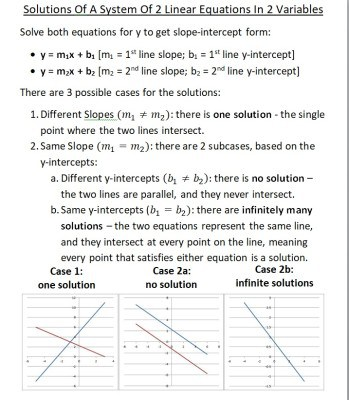A system of 2 linear equations in 2 variables has infinitely many solutions when the two lines have the same slope and the same y-intercept (that is, the two equations are equivalent and represent the same line, so they intersect at every point on the line).

A system of equations in 2, 3, or more variables can have infinite solutions.  We’ll start with linear equations in 2 variables with infinite solution.

## When Does A Linear System Have Infinite Solutions? (System Of Linear Equations In 2 Variables)

There are a few ways to tell when a linear system in two variables has infinite solutions:

• Solve the system – if you solve the system and get an equation that is always true, regardless of variable value (such as 1 = 1), then there are infinite solutions.
• Look at the graph – if the two lines are the same (they overlap, or intersect everywhere on the line), then there are infinite solutions to the system.
• Look at the slope and y-intercept – solve both equations for y to get slope-intercept form, y = mx + b.  If the two equations have the same slope and the same y-intercept, then the lines are equivalent and there are infinite solutions (you can get a refresher on how to tell when two lines are parallel in my article here).

We’ll look at some examples of each case, starting with solving the system.

### Solving A Linear System With Infinite Solutions

When we attempt to solve a linear system with infinite solutions, we will get an equation that is always true as a result.  For example, after we simplify and combine like terms, we will get something like 1 = 1 or 5 = 5.

Let’s take a look at some examples to see how this can happen.

#### Example 1: Using Elimination To Show A Linear System Has Infinite Solutions

Let’s say we want to solve the following system of linear equations:

• 2x + 4y = 3
• -6x – 12y = -9

We will use elimination to solve.  Let’s try to eliminate the “x” variable.

We begin by multiplying the first equation by 3 to get:

• 3(2x + 4y) = 3(3)  [multiply the first equation by 3 on both sides]
• 6x + 12y = 9  [distribute the 3 through parentheses]

Now we add this modified equation to the second one:

6x + 12y = 9

+

-6x – 12y = -9

___________

0x + y = 0

This implies 0 = 0, which is always true – regardless of the values of x or y we choose.  This means that both equations represent the same line.

It also means that every point on that line is a solution to this linear system.  So, the system has infinite solutions.

The graph below shows the line resulting from both of the equations in this system.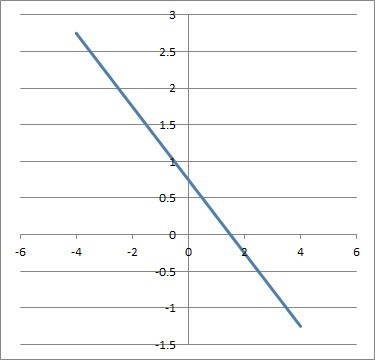The two equations 2x + 4y = 3 and -6x – 12y = -9 have the same slope and the same y-intercept, so they are the same line and they intersect at all points on the line, meaning there are infinite solutions to the linear system.

#### Example 2: Using Substitution To Show A Linear System Has Infinite Solutions

Let’s say we want to solve the following system of linear equations:

• y = 2x + 5
• 10x – 5y = -25

We will use substitution to solve.  We’ll substitute the y from the first equation into the y in the second equation:

• 10x – 5(2x + 5) = -25  [substitute y = 2x + 5 from the first equation]
• 10x – 10x – 25 = -25  [distribute 5 through parentheses]
• 0x = 0  [combine like terms on opposite sides]

This implies 0 = 0, which is always true – regardless of the values of x or y we choose.  This means that both equations represent the same line.

It also means that every point on that line is a solution to this linear system.  So, the system has infinite solutions.

The graph below shows the line resulting from both of the equations in this system.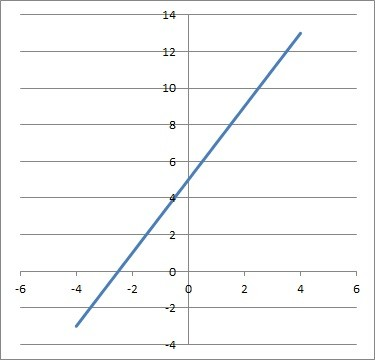The two equations y = 2x + 5 and 10x – 5y = -25 have the same slope and the same y-intercept, so they are the same line and they intersect at all points on the line, meaning there are infinite solutions to the linear system.

### Looking At The Graph Of A Linear System With Infinite Solutions

When we graph a linear system with infinite solutions, we will get two lines that overlap.  That is, they intersect at every point on the line, since the two equations are equivalent and give us the same line.

Let’s take a look at some examples to see how this can happen.

#### Example 1: Graph Of Two Equivalent Equations From A Linear System With Infinite Solutions

Let’s graph the following system of linear equations:

• y = 2x + 4
• -2y = -4x – 8

The lines have the same slope (m = 2) and the same y-intercept (b = 4), as you can see in the graph below: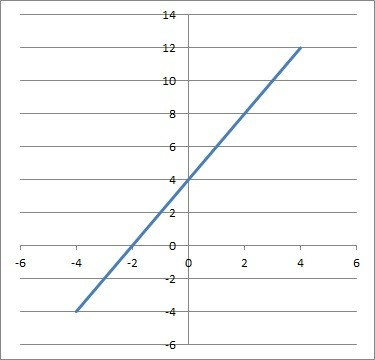The two equations y = 2x + 4 and -2y = -4x – 8 have the same slope and the same y-intercept, so they are the same line and they intersect at all points on the line, meaning there are infinite solutions to the linear system.

Since the slopes are the same and the y-intercepts are the same, the equations represent the same line.  So, they will intersect at every point on the line.

This means that there are infinite solutions to the linear system we started with.

#### Example 2: Graph Of Two Equivalent Equations From A Linear System With Infinite Solutions

Let’s graph the following system of linear equations:

• y = 4
• 3y = 12

The lines are horizontal, so they both have the same slope (m = 0).  They also have the same y-intercept (b = 4), as you can see in the graph below: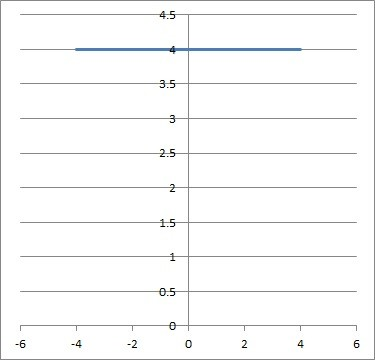The two equations y = 4 and 3y = 12 have the same slope and the same y-intercept, so they are the same line and they intersect at all points on the line, meaning there are infinite solutions to the linear system.

Since the slopes are the same and the y-intercepts are the same, the equations represent the same line.  So, they will intersect at every point on the line.

This means that there are infinite solutions to the linear system we started with.

Having math trouble?

Looking for a tutor?

### Looking At The Slope & Y-Intercept Of A Linear System With Infinite Solutions

When we solve a linear equation for y, we get slope-intercept form.  If we do this for both equations in a linear system, we can compare the slope and y-intercept.

If the two slopes are the same and the y-intercepts are the same, then the two lines are equivalent, meaning they intersect at all points on the line and there are infinite solutions to the linear system.

Let’s take a look at some examples to see how this can happen.

#### Example 1: Comparing Slope & Y-Intercept To Show There Are Infinite Solutions To A System Of Two Linear Equations

Let’s say we have the following system of linear equations:

• 4x = – 2y + 8
• 7y = -14x + 28

We will solve for y in both equations to get slope-intercept form, y = mx + b.

Solving the first equation for y, we get:

• 4x = – 2y + 8
• 4x + 2y = 8  [add 2y to both sides]
• 2y = -4x + 8  [subtract 4x from both sides]
• y = -2x + 4  [divide by 2 on both sides]

Solving the second equation for y, we get:

• 7y = -14x + 28
• y = -2x + 4  [divide by 7 on both sides]

So, the two equations in slope-intercept form are:

• y = -2x + 4
• y = -2x + 4

Since these two equations have the same slope (m = -2) and the same y-intercept (b = 4), we know that they represent the same line.

Since the lines intersect at all points on the line, there are infinite solutions to the system.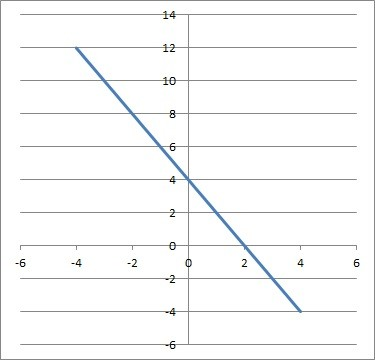The two equations 4x = -2y + 8 and 7y = -14x + 28 have the same slope and the same y-intercept, so they are the same line and they intersect at all points on the line, meaning there are infinite solutions to the linear system.

#### Example 2: Comparing Slope & Y-Intercept To Show There Are Infinite Solutions To A System Of Two Linear Equations

Let’s say we have the following system of linear equations:

• 30x = 6y – 18
• 4y – 20x + 10 = 22

We will solve for y in both equations to get slope-intercept form, y = mx + b.

Solving the first equation for y, we get:

• 30x = 6y – 18
• 30x + 18 = 6y  [add 18 to both sides]
• 5x + 3 = y  [divide by 6 on both sides]

Solving the second equation for y, we get:

• 4y – 20x + 10 = 22
• 4y – 20x = 22 – 10  [subtract 10 from both sides]
• 4y – 20x = 12  [combine like terms: constants]
• 4y = 20x + 12  [add 20x to both sides]
• y = 5x + 3  [divide by 4 on both sides]

So, the two equations in slope-intercept form are:

• y = 5x + 3
• y = 5x + 3

Since these two equations have the same slope (m = -2) and the same y-intercept (b = 4), we know that they represent the same line.

Since the lines intersect at all points on the line, there are infinite solutions to the system.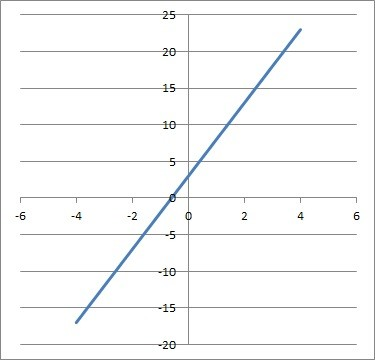The two equations 30x = 6y – 18 and 4y – 20x +10 = 22 have the same slope and the same y-intercept, so they are the same line and they intersect at all points on the line, meaning there are infinite solutions to the linear system.

## How To Create A System Of Linear Equations With Infinite Solutions

To create a system of linear equations with infinite solutions, we can use the following method:

• First, write a linear equation of the form ax + by = c.  Next, choose a nonzero number d.  Then, multiply both sides of the equation by d to get adx + bdy = cd.  This second equation is equivalent to the first, and we have our system.

### Example: Create A System Of Linear Equations With Infinite Solutions

First, we choose any values for a, b, and c that we wish.  This gives us our first equation:

• 2x + 5y = 9  [we chose a = 2, b = 5, c = 9]

Next, we choose a nonzero value of d: d = 4.

Now, we multiply both sides of the first equation by d = 4:

• 2x + 5y = 9  [first equation]
• 4(2x + 5y) = 4(9)  [multiply both sides by d = 4]
• 8x + 20y = 36  [distribute 4 through parentheses]

Now we have our second equation.

Our system of two equations is:

• 2x + 5y = 9
• 8x + 20y = 36

Since the two equations are equivalent, they represent the same line on a graph.  So, there are infinite solutions to this system.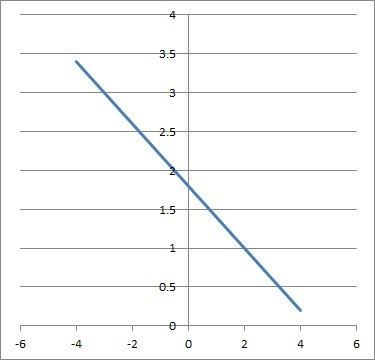The two equations 2x + 5y = 9 and 8x + 20y = 36 have the same slope and the same y-intercept, so they are the same line and they intersect at all points on the line, meaning there are infinite solutions to the linear system.

## System Of Linear Equations In Three Variables With Infinite Solutions

A system of equations in 3 variables will have infinite solutions if the planes intersect in an entire line or in an entire plane.

The latter case occurs if all three equations are equivalent and represent the same plane.

Here is an example of the second case:

• x + y + z = 1
• 2x + 2y + 2z = 2
• 3x + 3y + 3z = 3

Note that the second equation is the first equation multiplied by 2 on both sides.  Also note that the third equation is the first equation multiplied by 3 on both sides.

Since the equations are all multiples of one another, they are equivalent.  That means they all represent the same plane.

So, their intersection is the entire plane described by the equation x + y + z = 1.

This means that there are infinite solutions to the above system: every point on the plane x + y + z = 1.

## When Does A System Of Linear Equations Have A Solution?

A system of linear equations in two variables has a solution when the two lines intersect in at least one place.

• If the two lines have the same slope and the same y-intercept, then the two equations are equivalent, and they represent the same line (so there are infinitely many solutions, since every point on the line is a solution).
• If the two lines have different slopes, then they intersect at exactly one point.

## When Does A System Of Linear Equations Have No Solution?

A system of two linear equations in two variables has no solution when the two lines are parallel.

From an algebra standpoint, this means that we get a false equation when solving the system.

Visually, the lines never intersect on a graph, since they have the same slope but different y-intercepts.

## Conclusion

Now you know when a system of linear equations has infinite solutions.  You also know what to look out for in terms of the slope, y-intercept, and graph of lines in these systems.